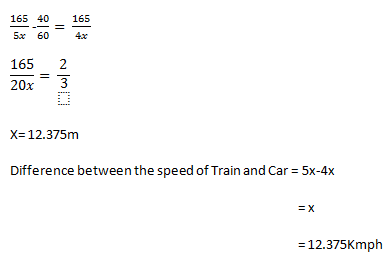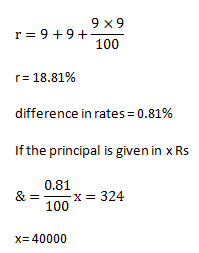# SSC Quantitative Aptitude Questions (Day-60)

Dear Aspirants, you can find the Quantitative Aptitude questions with detailed explanations for the SSC exams. Nowadays the competitive level of the exam has been increasing consistently. Due to the great demand for the government job, the level of the toughness reached greater. Candidates have to enhance the preparation process in order to drive in the right path. It doesn’t need to clear the prescribed cutoff. You must have to score good marks more than the cut off marks to get into the final provisional list. Here we have updating the Quantitative Aptitude questions with detailed explanations on a daily basis. You can practice with us and measure your level of preparation. According to that you can sculpt yourself in a proper way. SSC aspirants kindly make use of it and grab your success in your career.

Start Quiz

1) The ratio of the speed of Aman, Kamal and Manan is 4 : 5 : 6 respectively. What is the ratio of the time taken by Aman, Kamal and Manan respectively to cover the same distance?

(a) 10 : 12 : 15

(b) 6 : 5 : 4

(c) 4 : 5 : 6

(d) 15 : 12 : 10

2) Average of 36 results is 18. If 2 is subtracted from each result, then what will be the new average of the results?

(a) 9

(b) 16

(c) 17

(d) 17.5

3) The average marks obtained by 140 students in an examination is 90. If the average marks of passed students is 120 and the number of students who failed the examination are 90, then what is the average marks of students who failed the examination?

(a) 60

(b) 73.33

(c) 85.66

(d) 88

4) A shopkeeper professes to sell his goods at cost price but uses a 930 gm weight instead of 1 kilogram weight. What will be the proﬁt percentage of the shopkeeper?

(a) 3.76

(b) 7.52

(c) 9.85

(d) 10.36

5) The cost of pencil is 33
% less than the cost price of a pen. If a man sells 4 pencils at the cost price of 5 pens, the what is his profit percentage?

(a) 50

(b) 66.66

(c) 75

(d) 87.5

6) If the radius of a circle is increased by 50%, then what will be the percentage increase in the area of circle?

(a) 225

(b) 125

(c) 150

(d) 175

7) If the height of cylinder is increased by 35% and radius is increased by 10%, then what will be the percentage increase in curved surface area of cylinder?

(a) 46.5

(b) 45

(c) 48.5

(d) 49.7

8) A boat goes 2 km upstream and 3 km downstream in 20 minutes. It goes 7 km upstream and 2 km downstream in 53 minutes. What is speed (in km/h) of the boat in still water?

(a) 75/7

(b) 120/7

(c) 135/7

(d) 150/7

9) A train can travel 25% faster than a car. Both start from point A at the same time and reach point B 165 km away at the same time. On the way the train takes 40 minutes for stopping at the stations. What is the difference in the speeds (in km/hr) of train and car?

(a) 6.375

(b) 7.635

(c) 9.75

(d) 12.375

10) An amount was lent for one year at the rate of 18% per annum compounding annually. Had the compounding been done half yearly, the interest would have increased by 324. What was the amount (in Rs) lent?

(a) 32000

(b) 36000

(c) 40000

(d) 72000

Speed α 1/Time

Time α 1/Speed

Let the speed of Aman, Kamal and Manan is sa, sk, sm& time be ta,tk, tm

Henc,e

If Sa :Sk : Sm = 4 : 5 : 6

Then, ta :tk : tm = 1/4  :  1/5 : 1/6

Multiplying by 60.

= 15 : 12 : 10

If 2 is subtracted from each result then average will also decreased by 2. Hence new average will be = 18 – 2 = 16 yrs

Total = 140 × 90

= 12600

If 90 students failed then 50 student passed & total marks obtained by them.

= 50 × 120

= 6000

Total marks obtained by failed student = 12600 – 6000

Average of failed student = 6600/90 =  73.33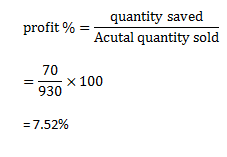pen/Pencil =3/2              (∵cost of pencil is 33.33% less than pen)

Assume the cost of pen i.e 3R

Cost pencil is 2.

CP = 4 × 2 = 8

SP = 5 × 3 = 15

% profit = (15 – 8)/8 × 100%

= 7/8 × 100 = 87.5%

Let the radius of the circle= r

50% increase the new radius be 3/2 r

Original area A = πr²

New area  A = 9/4 πr²

% increase in Area = (9/4-1) πr²/πr² × 100

= 5/4 × 100 = 125%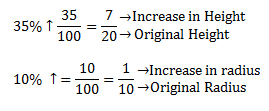Hence,

horiginal = 20

hnew= 27

roriginal = 10

rnew = 11

(CSA) original = 2πrh

= 2π ×20 ×10

= 400π

(CSA)new = 2π ×27 × 11

= 594π

% increase in (CSA) = 194/400 ×  100

= 48.5%

Let the upstream volume = 1/u

Downstream volume  = 1/v

2u + 3v = 20                        …(i)×2

7u + 2v = 53                        ….(ii)×3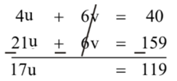u = 7

v = 2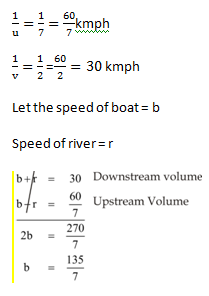Train : Car = 5:4

Let us suppose that the speed of train and car is 5x and 4x.